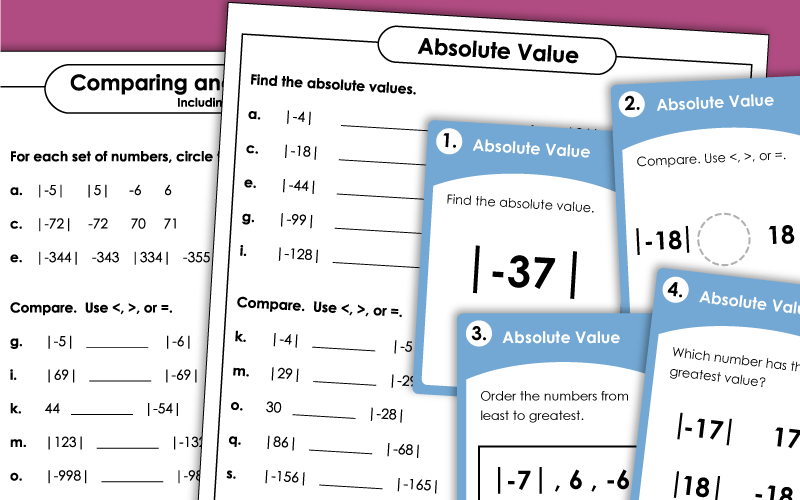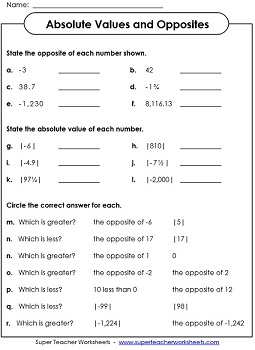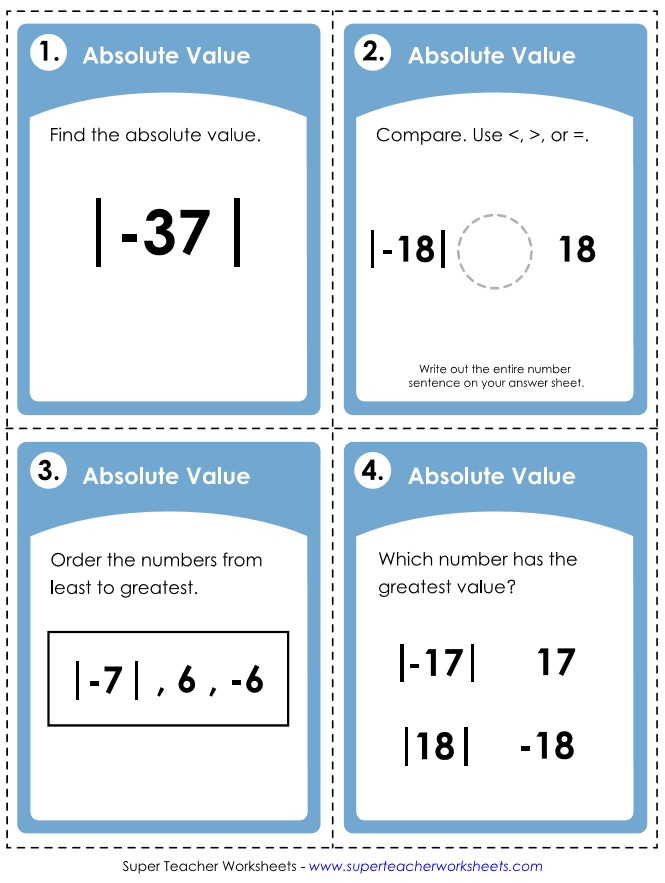# Absolute Value Worksheets

These printable worksheets can be used to teach your students about the absolute value of integers. Absolute value is simply the distance an integer is from zero on a number line. It is represented by two straight lines on either side of a number. For example, |-27| equals 27.## Absolute Value

Part 1: Find the absolute values. Part 2: Compare numbers with absolute values. Part 3: Find two values for each variable.
On this printable, students will compare pairs of numbers using <, >, and =. Then they will order sets of four numbers from least to greatest.
This file has 30 task cards. Use them for peer study groups, on your document camera for whole-group instruction, classroom scavenger hunts, or small group lessons.

## Absolute Value and Opposites

Find opposite numbers and absolute values. Then circle the correct answers to the math questions at the bottom of the page.
This worksheet has a series of questions about absolute and opposite values.
Positive and Negative Integers

Identify, add, subtract, multiply, and divide positive and negative integers.

## Worksheet ImagesMy Account
Site Information